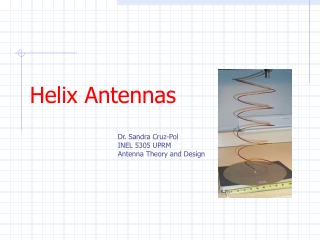DownloadDownload PresentationHelix Antennas

# Helix Antennas

Télécharger la présentation## Helix Antennas

- - - - - - - - - - - - - - - - - - - - - - - - - - - E N D - - - - - - - - - - - - - - - - - - - - - - - - - - -
##### Presentation Transcript

1. Helix Antennas Dr. Sandra Cruz-Pol INEL 5305 UPRM Antenna Theory and Design

2. Helix antennawas invented by J. D. Kraus. • There are two modes of operation, normal and axial. Axial Mode Normal Mode 3/4 l < C < 4/3 l NL<< l

3. not so Normal mode NL<< l • Pattern is (i) Si |Eq| = |Ef| tenemos polarización circular. (ii) En general la polarización será elíptica. (iii) Tiene un ancho de banda angosto debido a la dependencia en sus dimensiones geométricas. Normal Mode

4. Axial Mode 3/4 l < C < 4/3 l • Most widely used mode • Pattern has form: • where Axial Mode

5. Ns α Ground Plane: diameter>3l/4 Helix parameters • S = espaciamiento entre las vueltas • N = número de vueltas • a = ángulo de salida ("pitch angle") • C = circunferencia de cada vuelta = pD • D = diámetro • Dimensions that render an optimum pattern are : Para aparear la R, se puede aplastar el alambre a medida que se acerca al plano de la tierra ("ground plane") y se separa de este mediante un material dieléctrico

6. One loop in the helix…

7. Axial mode • Tiene mayor ancho de banda. • El patrón es más dirigido. • Se encuentra que a mayor número de vueltas, se obtiene mayor ganancia. • La impedancia de entrada de la espiral en este modo es casi toda real.

8. Design an end-fire right-hand circularly polarized helix having a half-power beamwidth of 45o , pitch angle of 13o , and a circumference of 60 cm at a frequency of 500 MHz. • Determine • turns needed • directivity • axial ration • lower and upper frequencies of the bandwidth over which the required parameters remain relatively constant • input impedance at the center frequency and the edges of the band from part d) • Answer: N=6, D=20.8 (13 dB), AR = 1.083, 375-667MHz, 140, 105, 187 W • 10.27 Design a helical antenna with a directivity of 15 dB that is operating in the axial mode and whose polarization is nearly circular. The spacing between the runs is l/10. • Determine the • number of turns • axial ratio, both as a dimensionless quantity and in dB • Directivity according to Krauss equation (in DB) • Answer: N=21, AR =1.02, HPBW= 36.8o D= 14.5dB or 15dB

9. 10.28 Design a 10 turn helical antenna so that at the center frequency of 10 GHz, the circumference of each turn is 0.95l. Assuming a pitch angle of 14o, determine the a. mode in which the antenna operates b. half-power beamwidth (degrees) c. directivity in dB. Answer: Axial mode, HPBW=36o , D=15dB

10. 10.29 A lossless 10-turn helical antenna with a circumference of one-wavelength is connected to a 78-ohm coaxial line, and it is used as a transmitting antenna in a 500 MHz spacecraft communication system. The spacing between turns is l/10. The power in the coaxial line from the transmitter is 5 watts. Assuming the antenna is lossless: a. what is radiated power? b. If the antenna were isotropic, what would the power density (W/m2) be at a distance of 10 km? c. What is the power density at the same distance when the transmitting antenna is a the 10-turn helix and the observation are made along the maximum of the major lobe? d. it at 10 – km along the maximum of the major lobe an identical 10-turn helix was placed as a receiving antenna, which was polarization-matched to the incoming wave, what is the maximum power (in watts) that can be received? Answer: Answer: R= 140 W, Prad=4.595W, Siso=3.656nW/m2, D=15, Shelix =54.8nW/m2, Ae=0.6m2, P­rec=26.6nW

11. Application • At Arecibo Observatory :some receivers work at • frequency range :300 MHz up to 6 GHz • plan to extend the range up to 10 GHz. • A good way to do a preliminary check to see if these receivers are working is by sending a test signal, circular or linear (vertical), depending on which receiver is being used.

12. Narrow Band Antennas Feed Horns Dipole Yagi-Uda Uniform Helical

13. Broad Band Antennas Array of narrow band antennas TaperedHelical Spiral Planar Tapered Yagi-Uda

14. At the Arecibo Observatory Some of their antennas look like these:

15. Polarization helical antennas have circular polarization

16. Parameters Varied Conductor material Size of conductor

17. Cont. Parameters Varied SMA connector Feeding point Tuning From Ing.Natalia Figueroa UPRM,Lisa S. Wray &Edgar Castro (Arecibo Obs),2001

18. Preliminary Tests S11 parameters tests where done on the Large_B antenna. These measures the return loss on the antenna, ideally we want –10 dB. Also a the Smith Chart results where plotted. Large_B From Ing.Natalia Figueroa UPRM,Lisa S. Wray &Edgar Castro (Arecibo Obs),2001